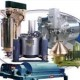## Chemical Reactor with Mass Transfer Limitations – in Comsol

In chemical reactors with a catalyst, sometimes the rate of reaction is governed by the concentration on/in the catalyst, which can be different from the concentration in the bulk fluid. This happens when the rate of mass transfer is not fast enough to get the material to the catalyst as fast as it is reacting. One cause is the boundary layer around the catalyst due to fluid flow. While that can be a complicated problem, we usually just lump everything together and define a mass transfer coefficient that defines the rate that the chemical passes through the boundary layer, usually depending on the difference between the concentration in the bulk stream and on the catalyst. Then one must solve an algebraic equation to find the concentration on the catalyst. This is done in the book using MATLAB, p. 155. It can be done in Comsol, too, using the feature to solve algebraic equations.  The latest addition to the book website, www.ChemEComp.com, shows how to do this. The example, while simple, shows how to solve ordinary differential equations as initial value problems along with an algebraic equation that must be satisfied at each point along the reactor. In this case, as we found with some of the mass balance problems, the initial guess of the variables defined by algebraic equations is important and some experimentation was necessary, but it was easily done.

Go to the book web site, www. ChemEComp.com and look at Download or chemical reactor with mass transfer.1.hari vishwakarma says:
•Makato says: